Question

# On the basis of kinetic theory, would you expect the rate of diffusion in a liquid...

On the basis of kinetic theory, would you expect the rate of diffusion in a liquid to increase or decrease as the temperature is increased? Explain by selecting all true statements.

1. The average distance traveled in a unit of time would decrease.

2. The average distance between collisions would stay about the same.

3. The average distance traveled in a unit of time would increase.

4.The average speed of the molecules would stay the same.

5. The average speed of the molecules would decrease.

6. The average speed of the molecules would increase.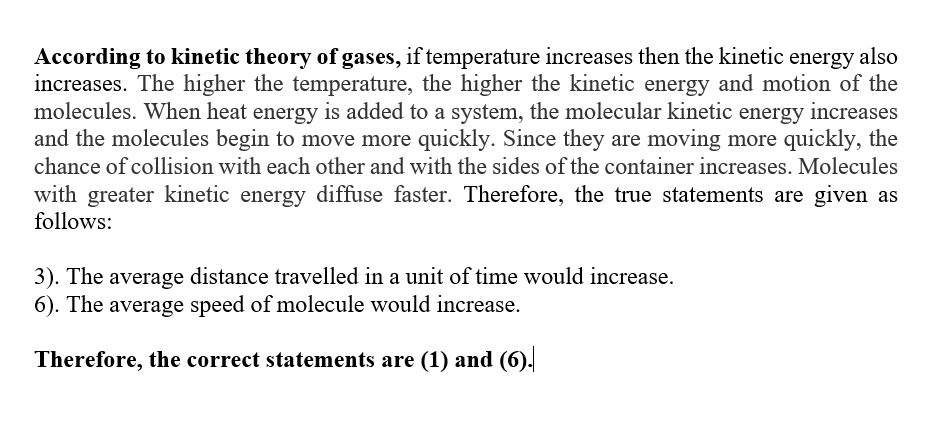#### Earn Coins

Coins can be redeemed for fabulous gifts.

Similar Homework Help Questions
• ### On the basis of kinetic theory, would you expect the rate of diffusion in a liguid...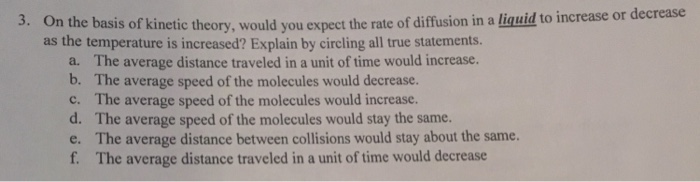On the basis of kinetic theory, would you expect the rate of diffusion in a liguid to increase or dec as the temperature is increased? Explain by circling all true statements. rease 3. The average distance traveled in a unit of time would increase. The average speed of the molecules would decrease. The average speed of the molecules would increase. The average speed of the molecules would stay the same. The average distance between collisions would stay about the same....

• ### According to the kinetic molecular theory of gases, which of the following gives rise to the...

According to the kinetic molecular theory of gases, which of the following gives rise to the pressure of a gas? Select one: a. the speed of individual molecules per unit time b. the average kinetic energy of molecules c. the total force due to collisions per unit area d. the presence of the gas in a specified volume at a particular temperature

• ### Foundations of Chemistry: 5th ed. another. The rate of diffusion depends on a. Based on the...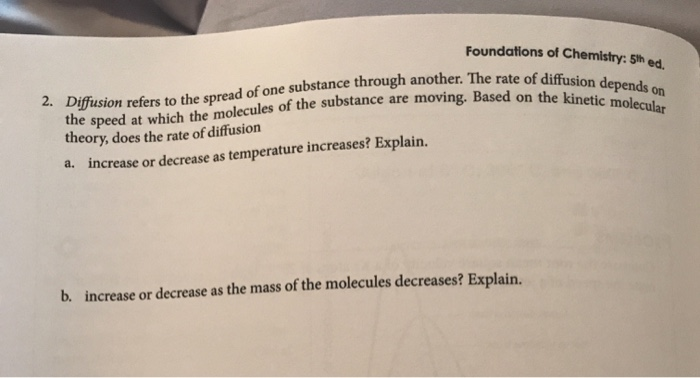Foundations of Chemistry: 5th ed. another. The rate of diffusion depends on a. Based on the kinetic molecular Diffusion refers to the spread of one substance through another. The the speed at which the molecules of the substance are moving. Based on th theory, does the rate of diffusion a. increase or decrease as temperature increases? Explain. b. increase or decrease as the mass of the molecules decreases? Explain. 4. The rate of diffusion of a particular gas was measured...

• ### 6. Use the kinetic molecular theory of gases to predict what would happen to a closed...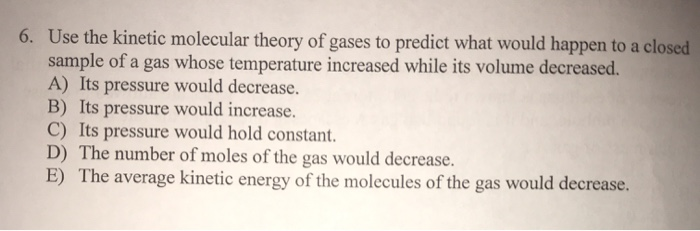6. Use the kinetic molecular theory of gases to predict what would happen to a closed sample of a gas whose temperature increased while its volume decreased. A) Its pressure would decrease. B) Its pressure would increase. C) Its pressure would hold constant. D) The number of moles of the gas would decrease. E) The average kinetic energy of the molecules of the gas would decrease.

• ### 1.) Two gases have the same temperature but different pressures. The kinetic molecular theory does not...

1.) Two gases have the same temperature but different pressures. The kinetic molecular theory does not predict that a. molecules in both gases have the same average kinetic energies. b. molecules in the low-pressure gas travel farther before they collide with other molecules. c. both gases have the same densities. d. all collisions of the molecules are elastic. 2.) On the basis of molecular structure and polarity, which of the following has the greatest solubity? a. CH4 b. CCl4 c....

• ### some context Problem 3: Use simple kinetic theory of gases discussed in section 1.3.2 as well...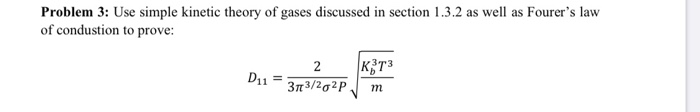some context Problem 3: Use simple kinetic theory of gases discussed in section 1.3.2 as well as Fourer's law of condustion to prove: 2 R373 D11 = 3113/202pm Dal We were unable to transcribe this imageof a nes. the xed the led negligible The following assumptions about the structure of the cases are made in order to investigate the statistical rules of the random motion of the molecules: The size of the gas molecules is negligible compared with the distance...

Please help with question, stuck on it. Please show all work of solution to comprehend it. 1.) In the simple kinetic theory of a gas, the molecules are assumed to be point-like objects (without any volume) so that they rarely collide with one another. In reality, each molecule has a small volume and so there are collisions. Let's assume that a molecule is a hard sphere of radius r. Then the molecules will occasionally collide with each other. The average...

• ### Which of the following is true about a 0.200 mol sample of nitrogen gas and a...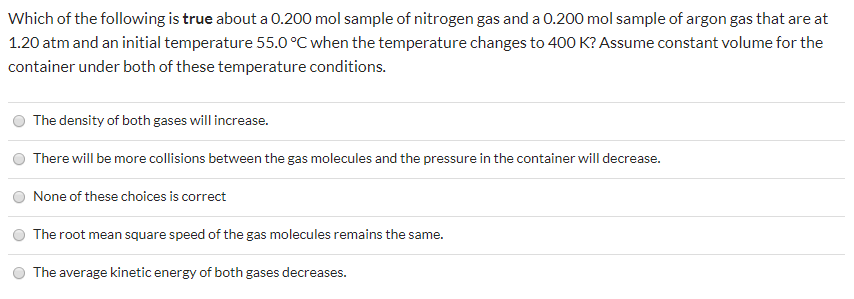Which of the following is true about a 0.200 mol sample of nitrogen gas and a 0.200 mol sample of argon gas that are at 1.20 atm and an initial temperature 55.0°C when the temperature changes to 400 K? Assume constant volume for the container under both of these temperature conditions. The density of both gases will increase. There will be more collisions between the gas molecules and the pressure in the container will decrease. None of these choices is...

• ### Question 29 (Mandatory) (1 point) Which of the following is true about the kinetic molecular theory?...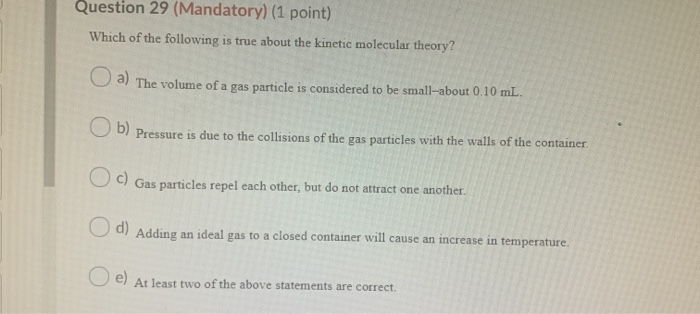Question 29 (Mandatory) (1 point) Which of the following is true about the kinetic molecular theory? U a) The volume of a gas particle is considered to be small-about 0.10 mL. U b) Pressure is due to the collisions of the gas particles with the walls of the container, U C) Gas particles repel each other, but do not attract one another. u d Adding an ideal gas to a closed container will cause an increase in temperature. u e)...

• ### if i increased the temperature for an experimental rate law problem, would variable in the rate...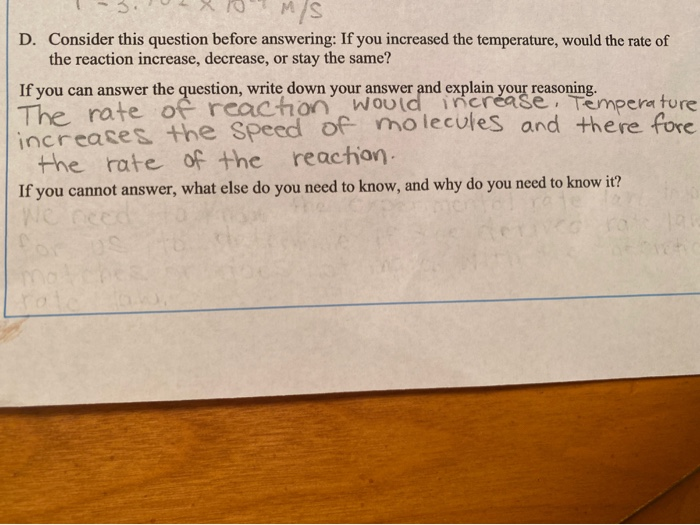if i increased the temperature for an experimental rate law problem, would variable in the rate law would be effected? is my answer correct, or is there some unknown variable? 1-3.02X10M/S D. Consider this question before answering: If you increased the temperature, would the rate of the reaction increase, decrease, or stay the same? If you can answer the question, write down your answer and explain your reasoning. The rate of reaction would increase, Temperature increases the speed of molecules...